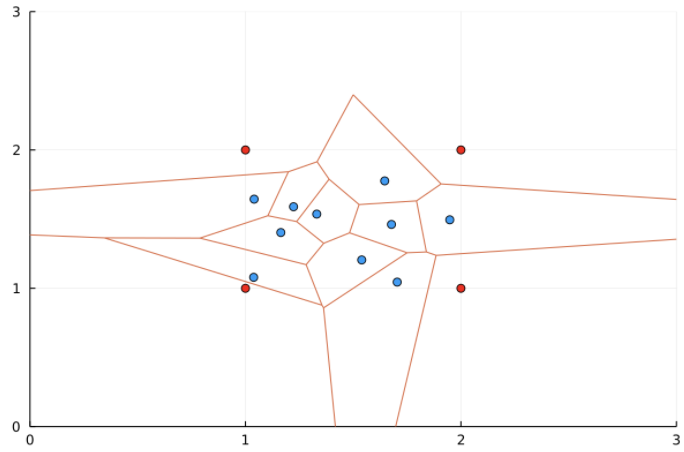# VoronoiDelaunay includes domain corners

The calculation of the Voronoi tessellation with the `VoronoiDelaunay.jl` package seems to add the bounding corners as points in the tessellation. Is there a way to turn this off? If the source code needs to be altered does anyone know where this feature is as I couldn’t figure it out?

``````using VoronoiDelaunay, Plots

N=10
x, y = rand(N).+1, rand(N).+1
tess = DelaunayTessellation()
push!(tess, Point2D.(x,y))

scatter(x,y, lims=(0,3), legend = false)
plot!(getplotxy(voronoiedges(tess))...)
scatter!((1,1), color=:red)
scatter!((1,2), color=:red)
scatter!((2,1), color=:red)
scatter!((2,2), color=:red)
``````Additionally, is there any way to achieve a tessellation with periodic boundaries?

Thank you very much for any pointers!